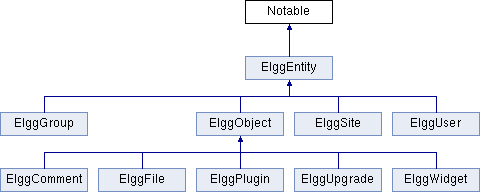Elgg  Version 1.11
Notable Interface Reference
Inheritance diagram for Notable:## Public Member Functions

setCalendarTimeAndDuration (\$hour=null, \$minute=null, \$second=null, \$day=null, \$month=null, \$year=null, \$duration=null)
Calendar functionality. More...

getCalendarStartTime ()
Return the start timestamp. More...

getCalendarEndTime ()
Return the end timestamp. More...

## Detailed Description

Definition at line 10 of file Notable.php.

## Member Function Documentation

 Notable::getCalendarEndTime ( )

Return the end timestamp.

Returns
int

Implemented in ElggEntity.

 Notable::getCalendarStartTime ( )

Return the start timestamp.

Returns
int

Implemented in ElggEntity.

 Notable::setCalendarTimeAndDuration ( \$hour = `null`, \$minute = `null`, \$second = `null`, \$day = `null`, \$month = `null`, \$year = `null`, \$duration = `null` )

Calendar functionality.

This function sets the time of an object on a calendar listing.

Parameters
 int \$hour If ommitted, now is assumed. int \$minute If ommitted, now is assumed. int \$second If ommitted, now is assumed. int \$day If ommitted, now is assumed. int \$month If ommitted, now is assumed. int \$year If ommitted, now is assumed. int \$duration Duration of event, remainder of the day is assumed.
Returns
bool

Implemented in ElggEntity.

The documentation for this interface was generated from the following file: